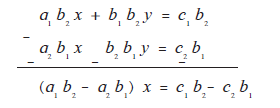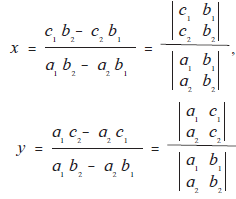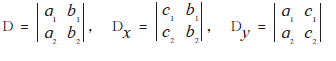Cramer'S Rule

notes

To use Cramer’s method, the equations are written as a1x + b1y = c1 and a2x + b2y = c2.

a_1x+b_1y=c_1   .............(I)

a_2x+b_2y=c_2  ..............(II)

Here x and y are variables, a1, b1, c1 and a2, b2, c2 are real numbers, a_1b_2-a_2b_1ne0

Now let us solve these equations.

Multiplying equation (I) by b2

a_1b_2x+b_1b_2y=c_1b_2   ............(III)

Multiplying equation (II) by b1.

a_2b_1x+b_2b_1y=c_2b_1  ..............(IV)

Subtracting equation (III) from (IV)x=(c_1b_2-c_2b_1)/(a_1b_2-a_2b_1) ...........(V)

Similarly y=(a_1c_2-a_2c_1)/(a_b_2-a_2b_1) ...........(V)

To remember and write the expressions

c_1b_2-c_2b_1, a_1b_2-a_2b_1, a_1c_2-a_2c_1 we use the determinants.

Now a_1x+b_1y=c_1

and a_2x+b_2y=c_2

We can write 3 columns ([a_1],[a_2]), ([b_1],[b_2]), ([c_1],[c_2])

The values x, y in equation (V), (VI) are written using determinants as followsTo remember let us denotetherefore x=D_x/D, y=D_y/D

For writting D, Dx, Dy remember the order of columns ([a_1],[a_2]), ([b_1],[b_2]), ([c_1],[c_2]) From the equations,

a_1x+b_1y=c_1

and a_2x+b_2y=c_2 We get the columns ([a_1],[a_2]), ([b_1],[b_2]), ([c_1],[c_2])

• In D the column of constants ([c_1],[c_2]) is omitted.
• In Dx the column of the coefficients of x, ([a_1],[a_2])  is replaced by ([c_1],[c_2]).
• In Dy the column of the coefficients of y, ([b_1],[b_2]) is replaced by  ([c_1],[c_2]).

definition

Using determinants, simultaneous equaions can be solved easily and in less space. This method is known as determinant method. This method was first given by a Swiss mathematician Gabriel Cramer, so it is also known as Cramer’s method.

If you would like to contribute notes or other learning material, please submit them using the button below.

Shaalaa.com

Cramer's Rule [00:10:39]
S
0%